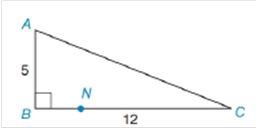Chapter 8.4, Problem 21EElementary Geometry For College St...

7th Edition
Alexander + 2 others
ISBN: 9781337614085

Solutions

Chapter
SectionElementary Geometry For College St...

7th Edition
Alexander + 2 others
ISBN: 9781337614085
Textbook Problem

Let N be any point on side BC of the right triangle ABC. Find the upper and lower limits for the length of AN.To determine

To Find:

The upper and lower limits for the length of AN.

Explanation

The given right triangle is

Here, AB=5 and BC=12.

Now find the length of AC of the right triangle.

According to Pythagoras theorem, AC2=AB2+BC2

Still sussing out bartleby?

Check out a sample textbook solution.

See a sample solution

The Solution to Your Study Problems

Bartleby provides explanations to thousands of textbook problems written by our experts, many with advanced degrees!

Get Started

In words, explain what is measured by SS, variance, and standard deviation.

Statistics for The Behavioral Sciences (MindTap Course List)

Evaluate the expression sin Exercises 116. 3(2)0

Finite Mathematics and Applied Calculus (MindTap Course List)

limx1x3x21x = _____. a) 1 b) 1 c) 0 d) does not exist

Study Guide for Stewart's Single Variable Calculus: Early Transcendentals, 8th

converges to: 2 the series diverges

Study Guide for Stewart's Multivariable Calculus, 8th